# NCERT Solutions for Class 9 Maths Exercise 13.5## myCBSEguide App

CBSE, NCERT, JEE Main, NEET-UG, NDA, Exam Papers, Question Bank, NCERT Solutions, Exemplars, Revision Notes, Free Videos, MCQ Tests & more.

NCERT Solutions for Class 9 Maths Exercise 13.5 book solutions are available in PDF format for free download. These ncert book chapter wise questions and answers are very helpful for CBSE board exam. CBSE recommends NCERT books and most of the questions in CBSE exam are asked from NCERT text books. Class 9 Maths chapter wise NCERT solution for Maths Book for all the chapters can be downloaded from our website and myCBSEguide mobile app for free.

NCERT solutions for Class 9 Maths Surface Areas and Volumes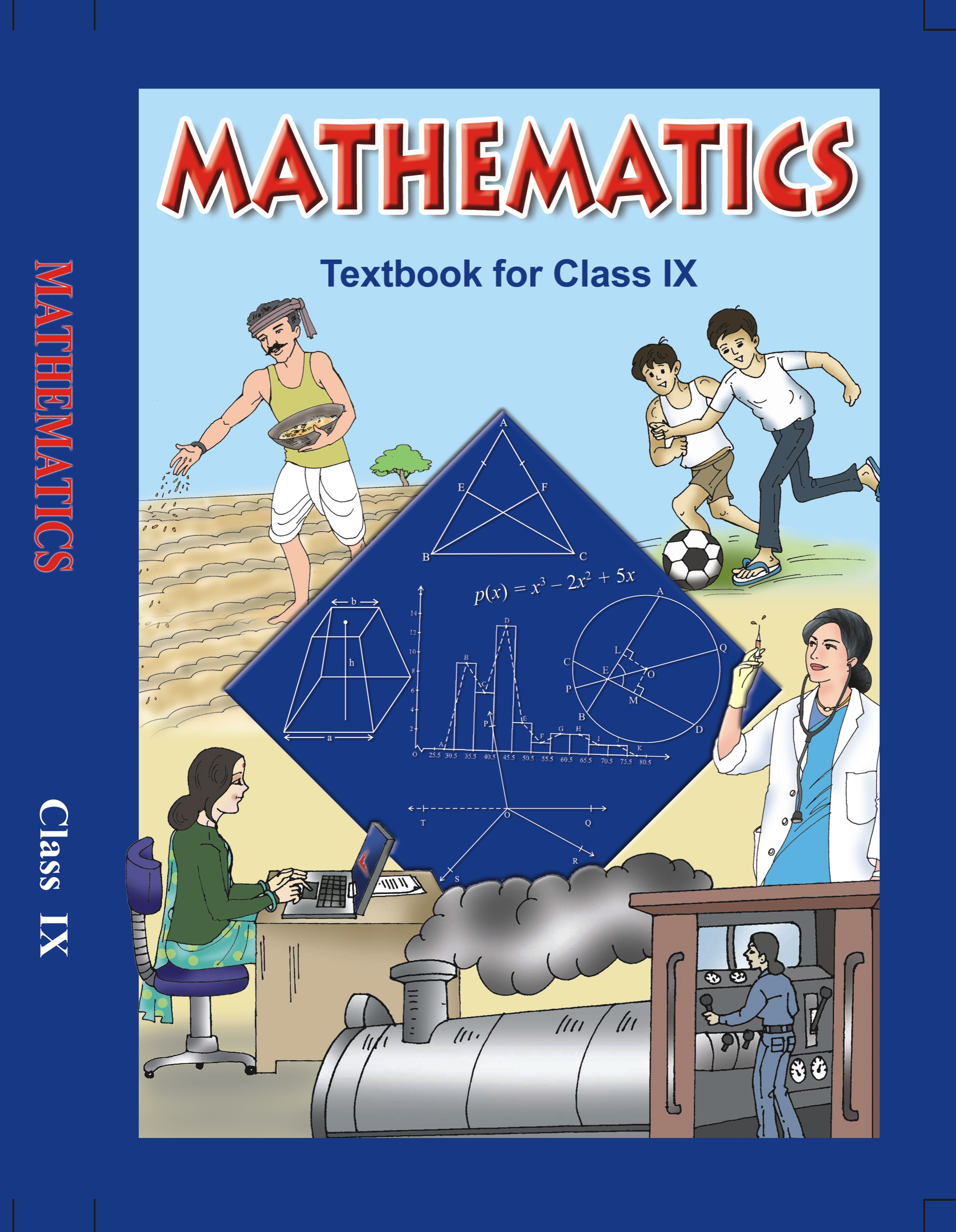## NCERT Solutions for Class 9 Mathematics Surface Areas and Volumes

###### 1. A matchbox 4 cm x 2.5 cm x 1.5 cm. What will be the volume a packet containing 12 such boxes?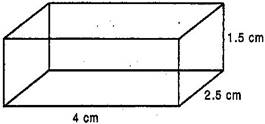Ans. Given: Length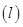= 4 cm, Breadth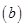= 2.5 cm, Height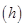= 1.5 cm

Volume of a matchbox =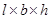= 4 x 2.5 x 1.5

= 15 cm3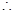Volume of a packet containing 12

such matchboxes = 12 x 15 = 180 cm3

NCERT Solutions for Class 9 Maths Exercise 13.5

###### 2. A cubical water tank is 6 m long, 5 m wide and 4.5 m deep. How many litres of water can it hold? (1 m3 = 1000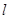)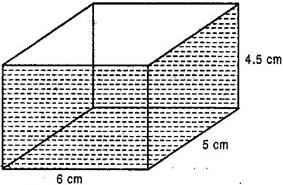Ans. Volume of water in cuboidal tank = 6 m x 5 m x 4.5 m

= 135 m3= 135 x 1000 liters

= 135000 liters

Hence tank can hold 135000 liters of water.

###### 3. A cuboidal vessel is 10 m long and 8 m wide. How high must it be to hold 380 cubic meters of a liquid?

Ans. Let height of cuboidal vessel =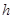m

Volume of liquid in cuboidal vessel

= 380 m3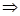= 380 cm310 m x 8 m x= 380=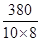= 4.75 m

Hence cuboidal vessel is 4.75 m high.

###### 4. Find the cost of digging a cuboidal pit 8 m long. 6 m broad and 3 m deep at the rate of Rs. 30 per m3.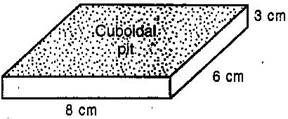Ans. Volume of cuboidal pit = 8 m x 6 m x 3 m= 144 m3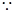Cost of digging 1 m3 cuboidal pit

= Rs. 30Cost of digging 144 m3 cuboidal pit

= 30 x 144 = Rs. 4320

NCERT Solutions for Class 9 Maths Exercise 13.5

###### 5. The capacity of a cuboidal tank is 50000 litres of water. Find the breadth of the tank, if its length and depth are respectively 2.5 m and 10 m.

(1 m3 = 1000)

Ans. Capacity of cuboidal tank

= 50000 liters= 50000 liters2.5 m x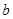x 10 m =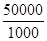m3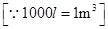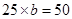= 2 m

Hence breadth of cuboidal tank is 2 m.

NCERT Solutions for Class 9 Maths Exercise 13.5

###### 6. A village having a population of 4000 requires 150 litres of water per head per day. It has a tank measuring 20 m by 15 m by 6 m. For how many days will the water of this tank last?

Ans. Capacity of cuboidal tank

== 20 m x 15 m x 6 m

= 1800 m3 = 1800 x 1000 liters= 1800000 liters

Water required by her head per day

= 150 liters

Water required by 4000 persons per day = 150 x 4000 = 600000 liters

Number of days the water will last

=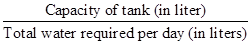=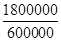= 3

Hence water of the given tank will last for 3 days.

NCERT Solutions for Class 9 Maths Exercise 13.5

###### 7. A godown measures 40 m x 25 m x 15 m. Find the maximum number of wooden crates each measuring 1.5 m x 1.25 m x 0.5 m that can be stored in the godown.

Ans. Capacity of cuboidal godown

= 40 m x 25 m x 15 m = 15000 m3

Capacity of wooden crate = 1.5 m x 1.25 m x 0.5 m = 0.9375 m3

Maximum number of crates that can be stored in the godown

=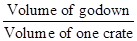=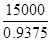= 16000

Hence maximum 16000 crates can be stored in the godown.

NCERT Solutions for Class 9 Maths Exercise 13.5

###### 8. Find the minimum number of bricks each measuring 22.5 cm x 11.5 cm x 7.5 cm required to construct a wall 10 m long, 6 m high and 1.5 m thick.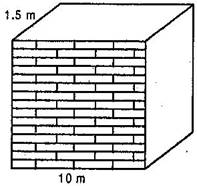Ans. Volume of one cuboidal brick

== 22.5 cm x 11. 5 cm x 7.5 cm3

= 1940.625 cm3

= 0.001940625 m3

Volume of cuboidal wal

= 10 m x 6 m x 1.5 m= 90 m3

Minimum number of bricks required

=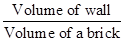=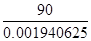=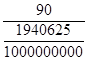=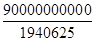= 46376.81

= 46377 [Since bricks cannot be in fraction]

NCERT Solutions for Class 9 Maths Exercise 13.5

###### 9. A solid cube of side 12 cm is cut into eight cubes of equal volume. What will be the side of the new cube? Also, find the ratio between their surface areas.

Ans. Volume of solid cube =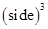=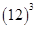= 1728 cm3

According to question, Volume of each new cube=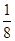(Volume of original cube)

=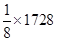= 216 cm3Side of new cube =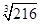= 6 cm

Now, Surface area of original solid cube = 6 (side)2

= 6 x 12 x 12 = 864 cm2

Now, Surface area of original solid cube = 6 (side)2= 6 x 6 x 6 = 216 cm2

Now according to the question,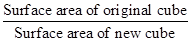=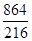=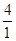Hence required ration between surface area of original cube to that of new cube = 4 : 1.

NCERT Solutions for Class 9 Maths Exercise 13.5

###### 10. A river 3 m deep and 40 m wide is flowing at the rate of 2 km per hour. How much water eill fall into the sea in a minute?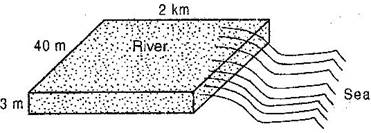Ans. Since water flows at the rate of 2 km per hour, the water from 2 km of the river flows into the sea in one hour.

Therefore, the volume of water flowing into the sea in one hour

= Volume of a cuboid

== 2000 m x 40 m x 3 m

= 240000 m3 [1 km = 1000 m]

Now, Volume of water flowing into sea in 1 hour (in 60 minutes)

= 240000 m3Volume of water flowing into sea in 1 minute =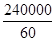= 4000 m3

NCERT Solutions for Class 9 Maths Exercise 13.5

###### 11. Find the length of a wooden plank of width 2.5 m, thickness 0.025 m and volume 0.25 m3.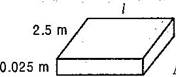Ans. Given: Volume of wooden plank

= 0.25 m3x 2.5 x 0.025 = 0.25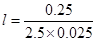= 4 m

Hence required length of wooden plank is 4 m.

## NCERT Solutions for Class 9 Maths Exercise 13.5

NCERT Solutions for Class 9 Maths PDF (Download) Free from myCBSEguide app and myCBSEguide website. Ncert solution class 9 Maths includes text book solutions from Mathematics Book. NCERT Solutions for CBSE Class 9 Maths have total 15 chapters. 9 Maths NCERT Solutions in PDF for free Download on our website. Ncert Maths class 9 solutions PDF and Maths ncert class 9 PDF solutions with latest modifications and as per the latest CBSE syllabus are only available in myCBSEguide.

## CBSE app for Class 9

To download NCERT Solutions for Class 9 Maths, Computer Science, Home Science,Hindi ,English, Social Science do check myCBSEguide app or website. myCBSEguide provides sample papers with solution, test papers for chapter-wise practice, NCERT solutions, NCERT Exemplar solutions, quick revision notes for ready reference, CBSE guess papers and CBSE important question papers. Sample Paper all are made available through the best app for CBSE students and myCBSEguide website.### 14 thoughts on “NCERT Solutions for Class 9 Maths Exercise 13.5”

1. I love this

2. Nice solutions.This solution. is very helpful for me .

3. Its very helpful this app is very good ..?????
I am very happy for this….???????????

4. Woooo…. its good hmmn…………. ????????

5. Ok

6. I am not getting my answer

7. Sooooo good app

8. Thank you so much

9. Thanks

10. wow awsome

11. It’s all and very helpful

12. Very good answer

13. Thanks allot for help

14. Thank you so so much